# Motion in One Dimension: JEE Advance(Part- 4) - Physics, Solution by DC Pandey Notes | Study DC Pandey Solutions for JEE Physics - JEE

## JEE: Motion in One Dimension: JEE Advance(Part- 4) - Physics, Solution by DC Pandey Notes | Study DC Pandey Solutions for JEE Physics - JEE

The document Motion in One Dimension: JEE Advance(Part- 4) - Physics, Solution by DC Pandey Notes | Study DC Pandey Solutions for JEE Physics - JEE is a part of the JEE Course DC Pandey Solutions for JEE Physics.
All you need of JEE at this link: JEE

Match the Columns

Ques 1: Match the following two columns.

 Column I Column II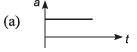(p) speed must be increasing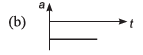(q) speed must be decreasing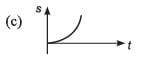(r) speed may be increasing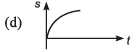(s) speed may be decreasing

Ans: (a) → r,s (b) →  r,s (c) →  p (d) →  q
Sol:
(a)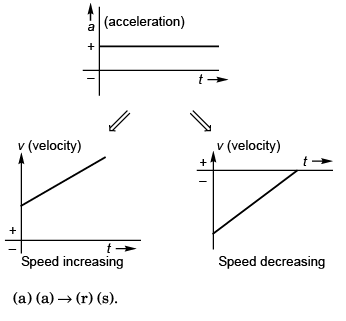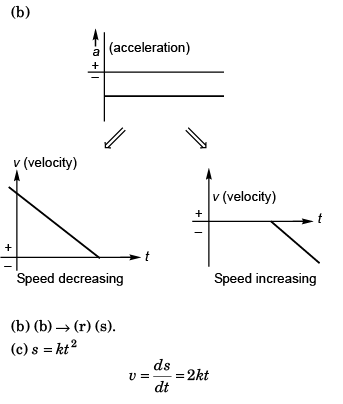∴ v must be increasing with time.
⇒ speed must also be increasing with time.
(c) → (p).
(d) Slope is + ive and decreasing.
∴ Velocity must be decreasing with time.
⇒ speed must also be decreasing with time. (d) → (q).

Ques 2: Match the following two columns.

 Column I Column II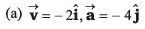(p) speed increasing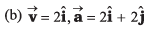(q) speed decreasing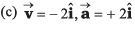(r) speed constant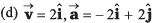(s) Nothing can be said

Ans: (a) → P (b) → p (c) → q (d) → q
Sol: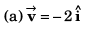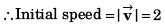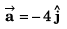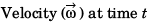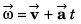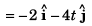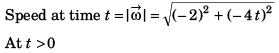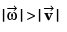Speed increasing.
∴ (a) → (p).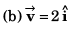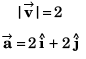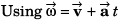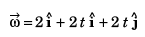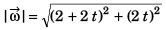As t > 0,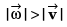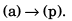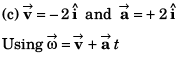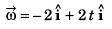or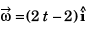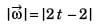At t = 0 s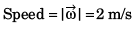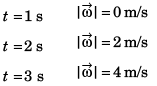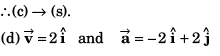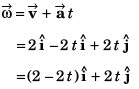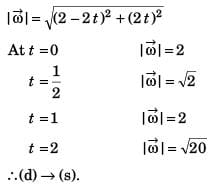Ques 3: The velocity-time graph of a particle moving along X-axis is shown in figure. Match the entries of Column I with entries of Column II.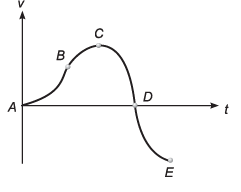Column I Column II (a) For AB, particle is (p) Moving in +ve X-direction with increasing speed (b) For BC, particle is (q) Moving in +ve X-direction with decreasing speed (c) For CD, particle is (r) Moving in -ve X-direction with increasing speed (d) For ZXE, particle is (s) Moving in +ve X-direction with decreasing speed

Ans: (a) → P (b) → p (c) → q (d) → r
Sol: (a) From A to B, v is increasing and area is + ive.
∴ (a) → (p).
(b) From B to C, v is increasing and area is + ive.
∴ (b) → (p).
(c) From C to D, v is decreasing while area is + ive.
∴ (c) → (q).
(d) From D to E, v is - ive and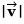is
increasing, area is -ive.
∴ (d) → (r).

Ques 4: Corresponding to velocity-time graph in one dimensional motion of a particle is shown in figure, match the following two columns.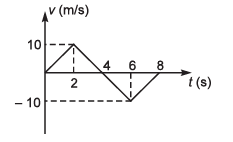Column I Column II (a) Average velocity between zero sec and 4 s (p) 10 SI units (b) Average acceleration between 1 s and 4 s (q) 2.5 SI units (c) Average speed between zero (r) 5 SI units (d) Rate of change of speed at 4s (s) None

Ans: (a) → (r) (b) → (s) (c) → (r) (d) → (r)
Sol: (a) Displacement = Area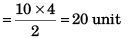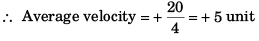i.e., (a) → (r).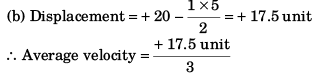= 5.83 unit
∴(b) → (s)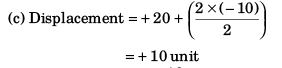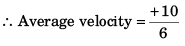∴ (c) → (s)
(d) Rate of change of velocity at t = 4 s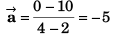∴ Rate of change of speed at
t = 4 s would be 5.
i.e., (d) ∴ (r).

Ques 5: A particle is moving along x-axis. Its x-coordinate varies with time as: x = -20 + 5t2
For the given equation match the following two columns

 Column I Column II (a) Particle will cross the origin at (p) zero sec (b) At what time velocity and acceleration are equal (q) 1 s (c) At what time particle changes its direction of motion (r) 2 s (d) At what time velocity is zero (s) None

Ans: (a) → (r) (b) → (q) (c) → (s) (d) → (p)
Sol: (a) x = - 20 + 5t2
0 = - 20 + 5t2
⇒ t = 2 s
i.e., (a) → (r).
(b) x = - 20 + 5t2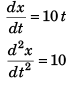Velocity will be numerically equal to acceleration at t = 1 s.
Thus (b) → (q).
(c) At t = 0 s   x = - 20 m
t = 1 s              x = - 15 m
t = 2 s             x = 0 m
t = 3 s             x = + 25 m
Particle is always moving along + ive x-axis.
∴ (c) → (s).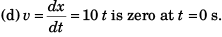∴ (d) → (p).

Ques 6: x and y-coordinates of particle movingin x - y plane are,
x = 1 - 2t + t2 and y = 4 - 4t + t2
For the given situation match the following two columns.

 Column I Column II (a) y - component of velocity when it crosses the y-axis (p) + 2 SI unit (h) x - component of velocity when it crosses the x-axis (q) - 2 SI units (c) Initial velocity of particle (r) + 4 SI units (d) Initial acceleration of particle (s) None

Ans: (a) → (q) (b) → (p) (c) → (s) (d) → (s)
Sol: (a) x = 1 - 2t + t2
0 = 1 - 2t + t2 [for particle to cross y-axis]
i.e., t = 1 s
Y = 4 - 4t + t2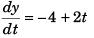= - 4 + (2 x 1) [at t = 1 s]
= - 2 unit
∴ (a) → (q).
(b) Y = 4 - 4t + t2
0 = 4 - 4t + t2 [For particle to cross x-axis]
i.e., t = 2 s
x = 1 - 2t + t2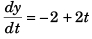= - 2 + (2 x 2) [at t = 2 s]
= + 2 unit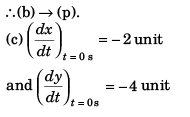∴  Initial velocity of particle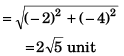i.e., (c) → (s).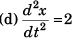and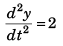∴   Initial acceleration of particle = 2√2 unit
i.e., (d) → (s).

The document Motion in One Dimension: JEE Advance(Part- 4) - Physics, Solution by DC Pandey Notes | Study DC Pandey Solutions for JEE Physics - JEE is a part of the JEE Course DC Pandey Solutions for JEE Physics.
All you need of JEE at this link: JEEUse Code STAYHOME200 and get INR 200 additional OFF

## DC Pandey Solutions for JEE Physics

210 docs

Track your progress, build streaks, highlight & save important lessons and more!

,

,

,

,

,

,

,

,

,

,

,

,

,

,

,

,

,

,

,

,

,

,

,

,

;